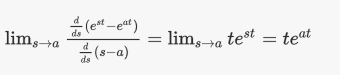$\frac { d y } { d t } = a y + e ^ { s t }$ $y = y(0) at t = 0$ Look for $y_p = Ye^{st}$

null solution的意思是，输入项没有了，也就是$e^{st} = 0$，求$\frac { d y } { d t } = a y$，得$y = e^{at}$

$y(t) = \frac{e^{st}}{s-a} + Ce^{at} = y_p + y_n\tag{1}$

y_p意思是y_particular，y_n意思是y_null。

$y(0) = \frac {1}{s-a} + C$

\begin{aligned} y ( t ) &\left. = \frac { e ^ { s t } } { s - a } + \left[ y ( 0 \right) - \frac { 1 } { s - a } \right] e ^ { a t } \ & = \frac { e ^ { s t - e ^ { a t } } } { s a } + y ( 0 ) e ^ { a t } \ & = y_{vp} + y_n \end{aligned}

y_vp是y_very particular。

IF s = a，then

$y_{vp}+y_n = t e^{at} + y(0)e^{at}\tag{2}$